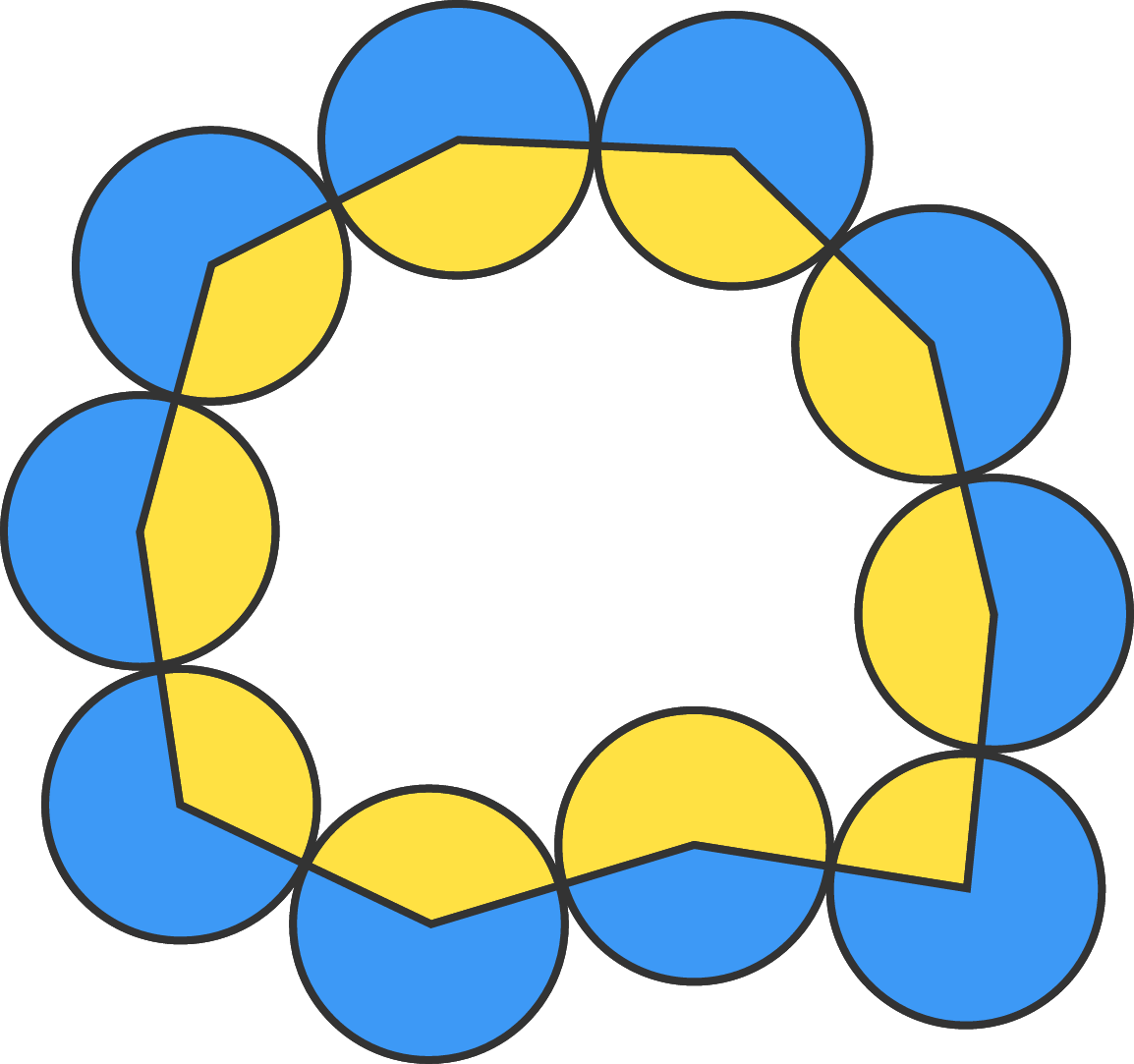# A necklace of circles

Geometry Level 210 identical circles of radius 2 are externally tangential to one another, as shown.

Connecting the centers of adjacent circles gives a 10-sided polygon, which divides the circles into two areas: blue and yellow. The positive difference between the blue and yellow areas is $k \pi.$

What is $k?$

×